TCGA数据库生存分析的网页工具哪家强

oncoln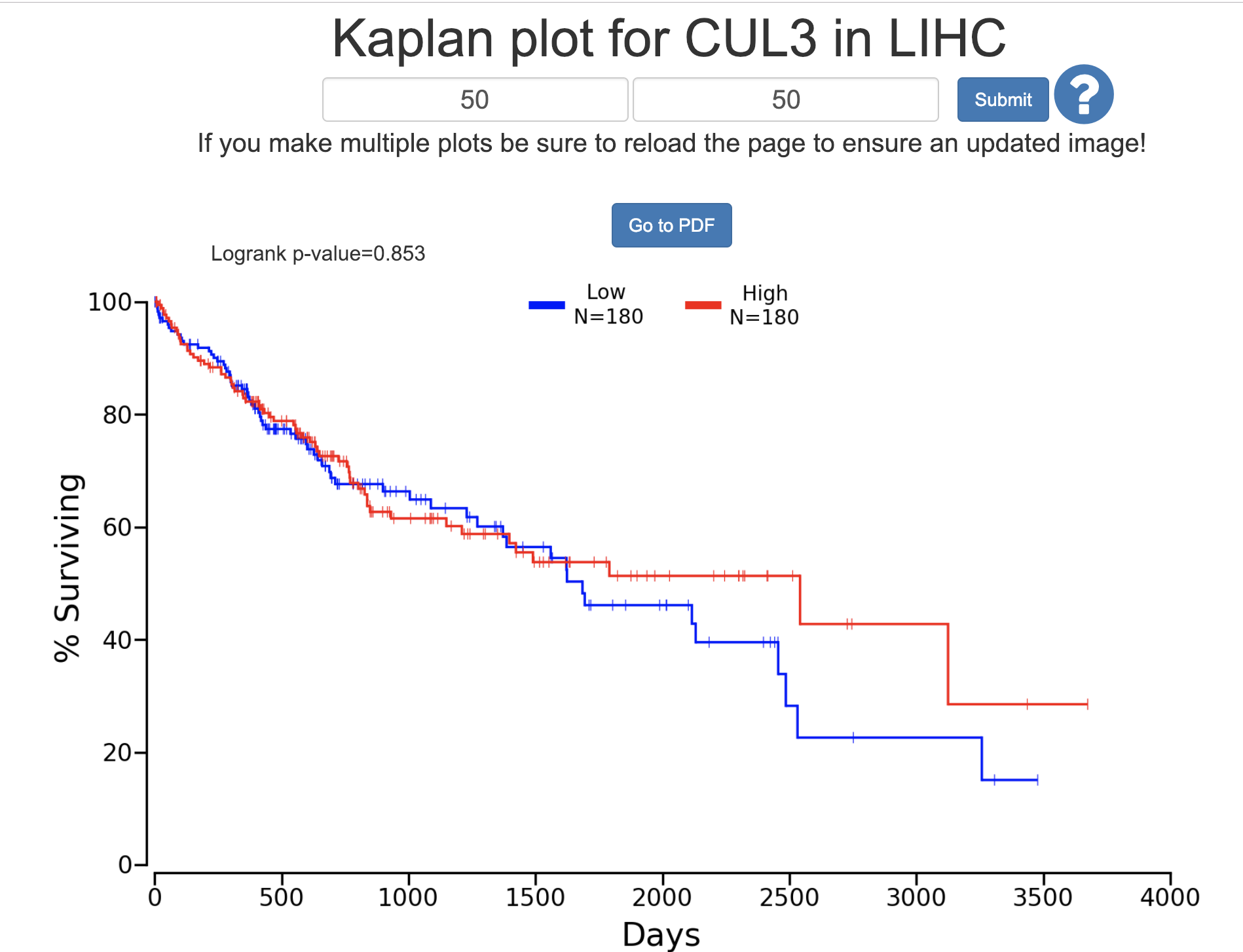kmplot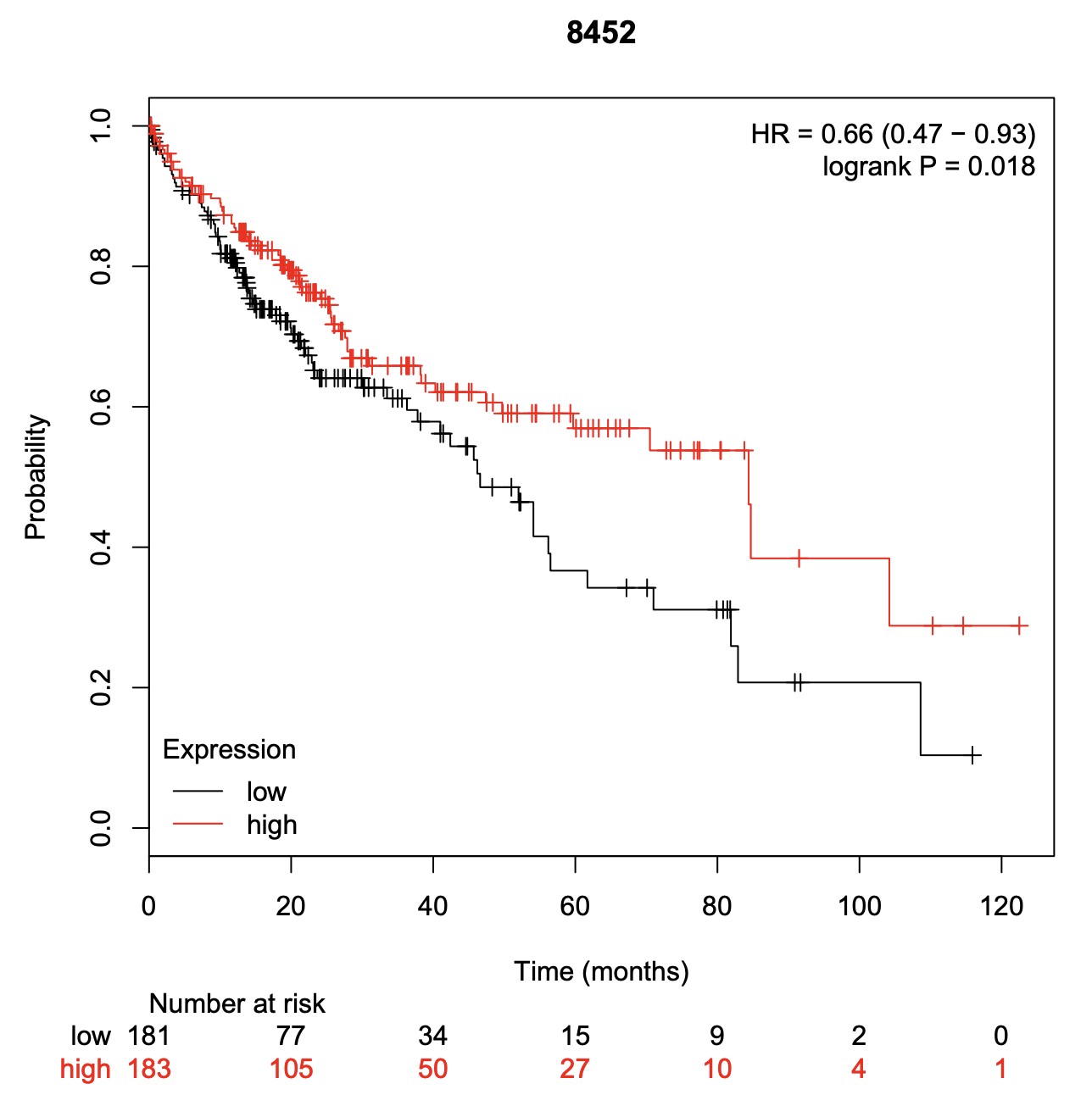GEPIA

• 总生存（Overall survival，OS）定义为：从随机化开始至（因任何原因）死亡的时间。被认为是肿瘤临床试验中最佳的疗效终点，当患者的生存期能充分时，它通常是首选终点。
• 无病生存期（Disease-free survival，DFS）定义为：从随机化开始至疾病复发或（因任何原因）死亡之间的时间。DFS 最常用于根治性手术或放疗后的辅助治疗的研究，目前是乳腺癌辅助性激素治疗、结肠癌辅助治疗、以及乳腺癌的辅助化疗的主要审批基础。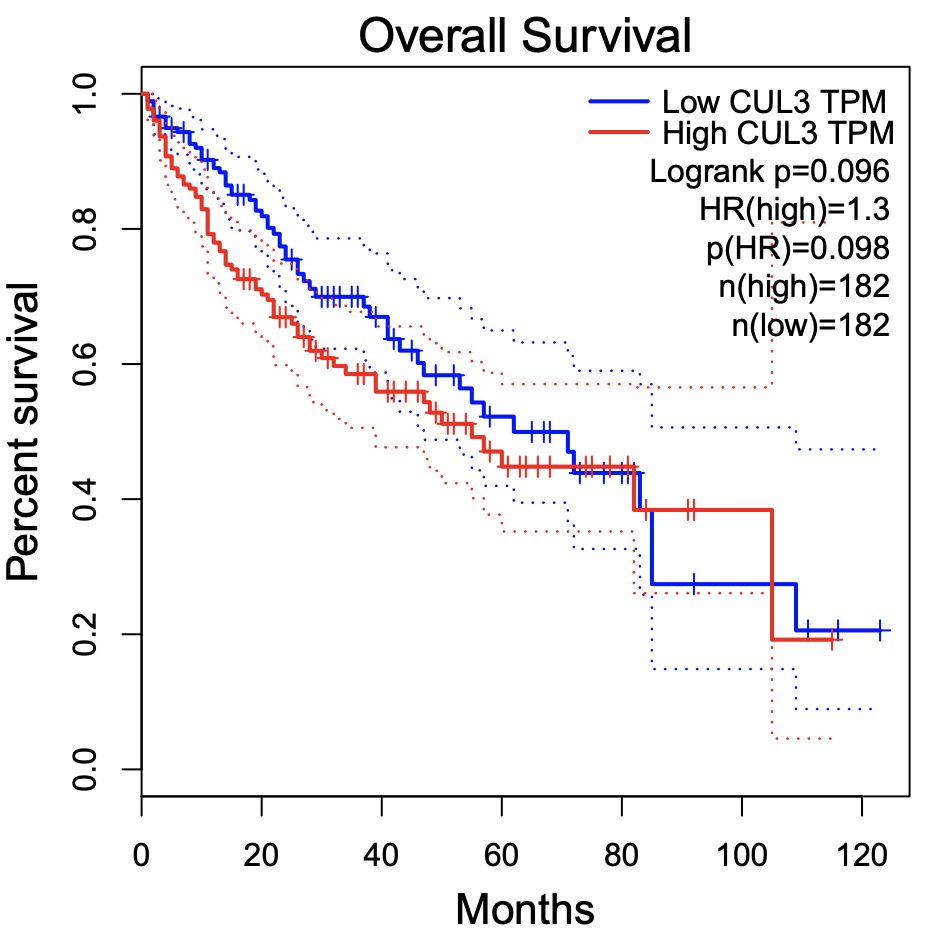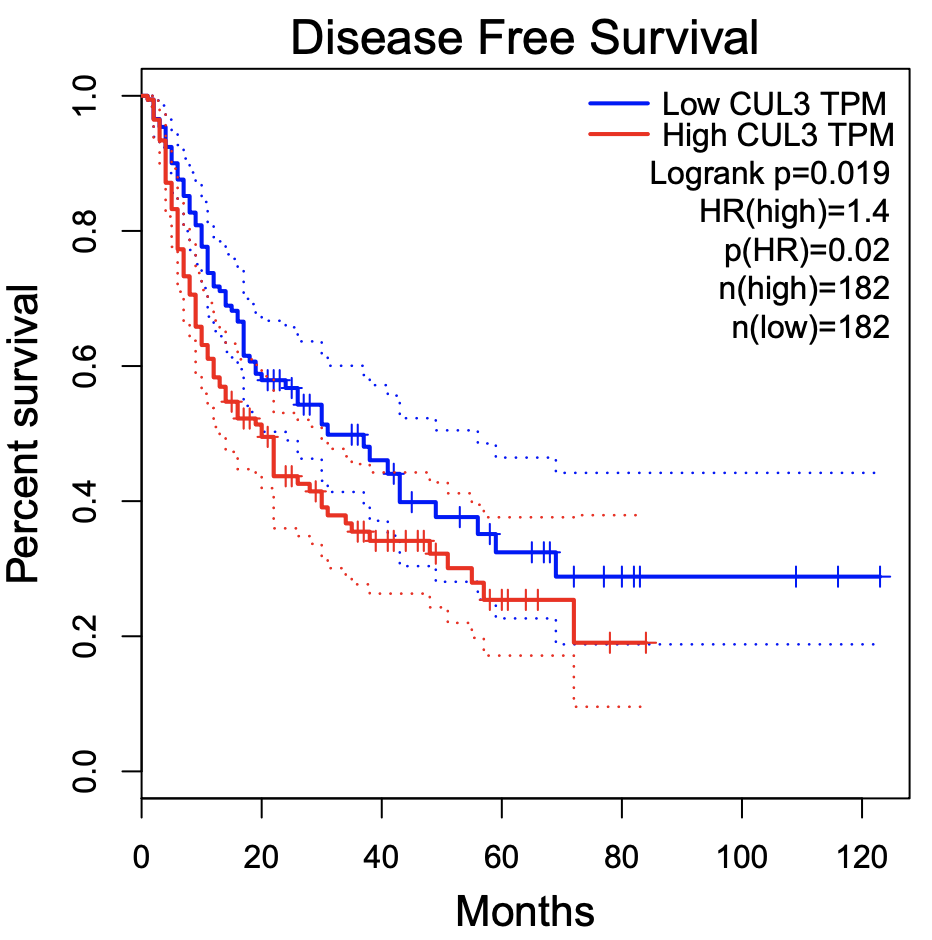在R里面重新画oncolnc数据

``````rm(list=ls())
options(stringsAsFactors = F)
# http://www.oncolnc.org/kaplan/?lower=20&upper=20&cancer=LIHC&gene_id=8452&raw=CUL3&species=mRNA
f='LIHC_8452_50_50.csv'
header = T,sep = ',',fill = T)
colnames(a)
dat=a
colnames(dat)
library(ggstatsplot)
ggbetweenstats(data =dat,
x = Group, y = 'Expression')

ggbetweenstats(data =dat,
x = Status, y = 'Expression')

library(ggplot2)
library(survival)
library(survminer)
table(dat\$Status)
sfit <- survfit(Surv(Days, Status)~Group, data=dat)
sfit
summary(sfit)
ggsurvplot(sfit, conf.int=F, pval=TRUE)
``````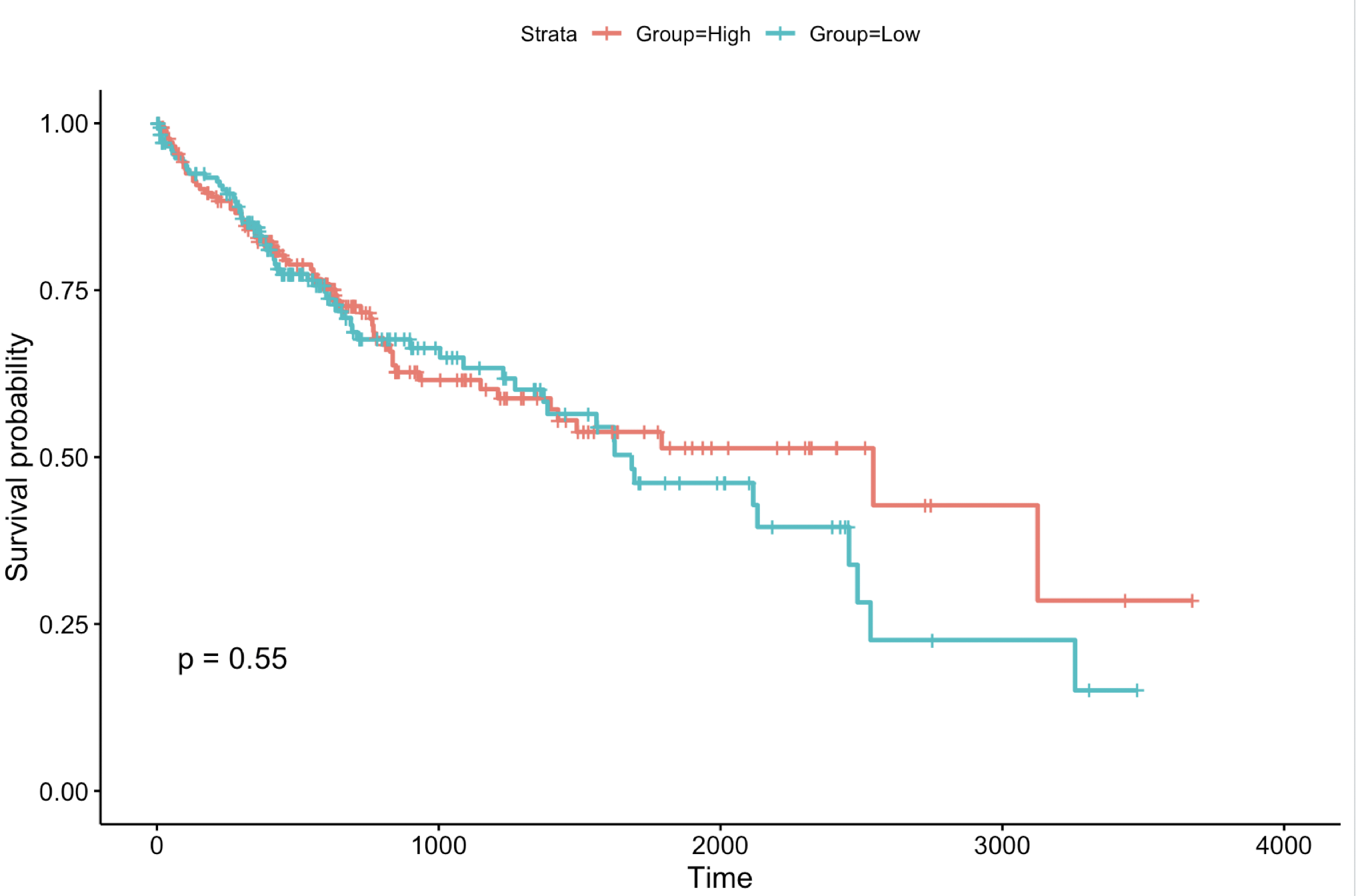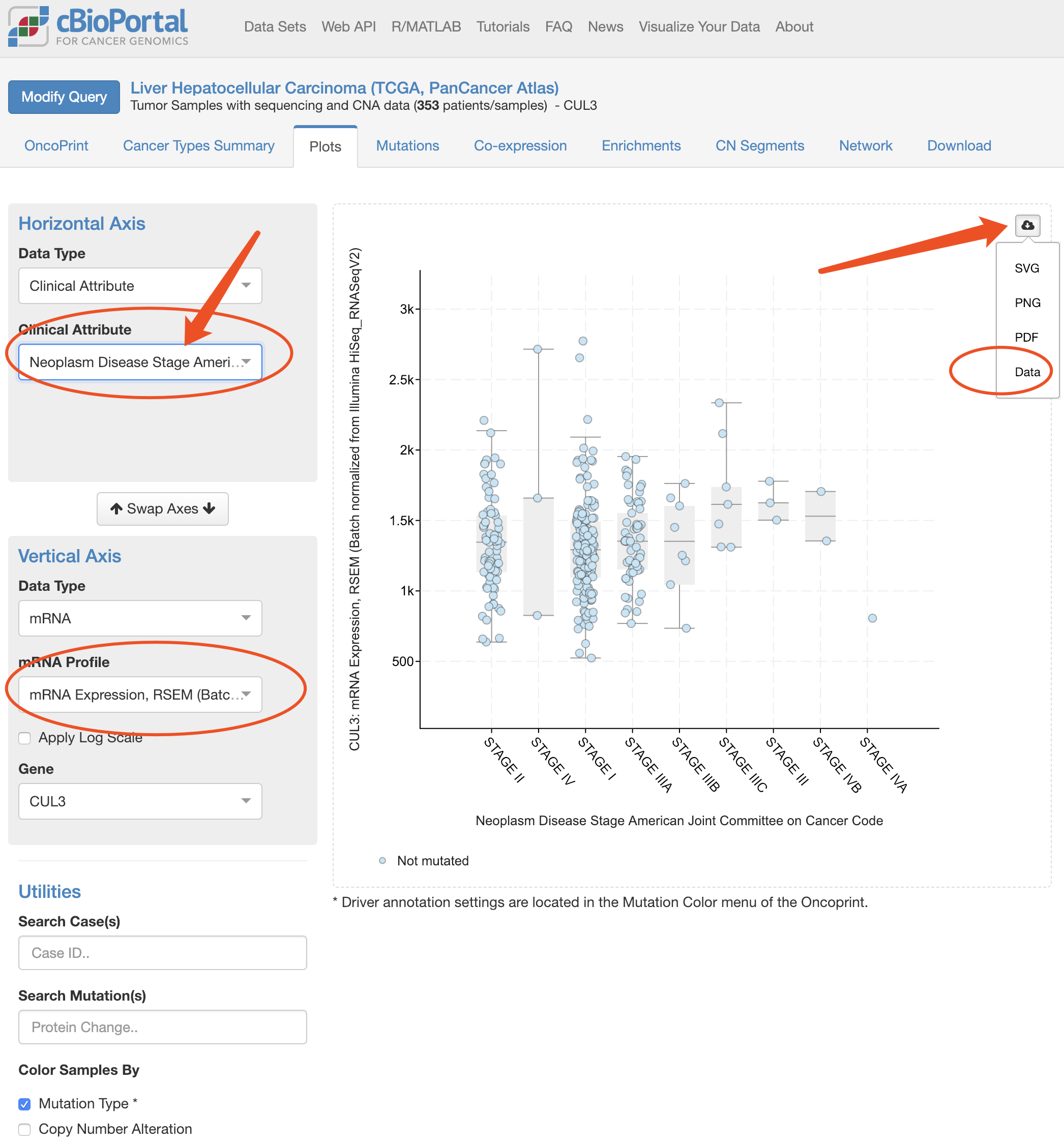``````rm(list=ls())
options(stringsAsFactors = F)
# http://www.oncolnc.org/kaplan/?lower=50&upper=50&cancer=LGG&gene_id=93663&raw=ARHGAP18&species=mRNA

# http://www.oncolnc.org
f='LIHC_8452_50_50.csv' ##
header = T,sep = ',',fill = T)
colnames(a)
dat=a
colnames(dat)
## http://www.cbioportal.org
header = T,sep = '\t',fill = T)
colnames(b)=c("Patient","stage","rsem")
b[,1]=substring(b[,1],1,12)
d=merge(a,b,by='Patient')

# https://xenabrowser.net/datapages/